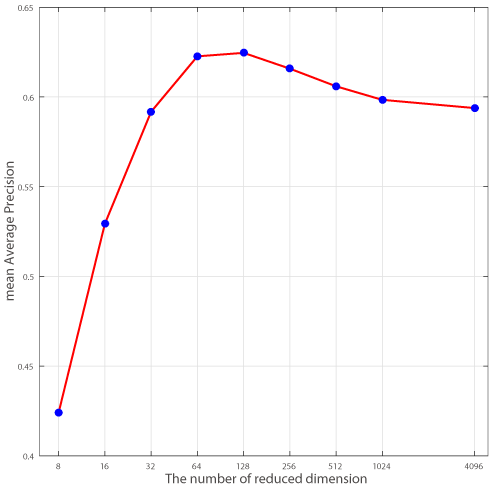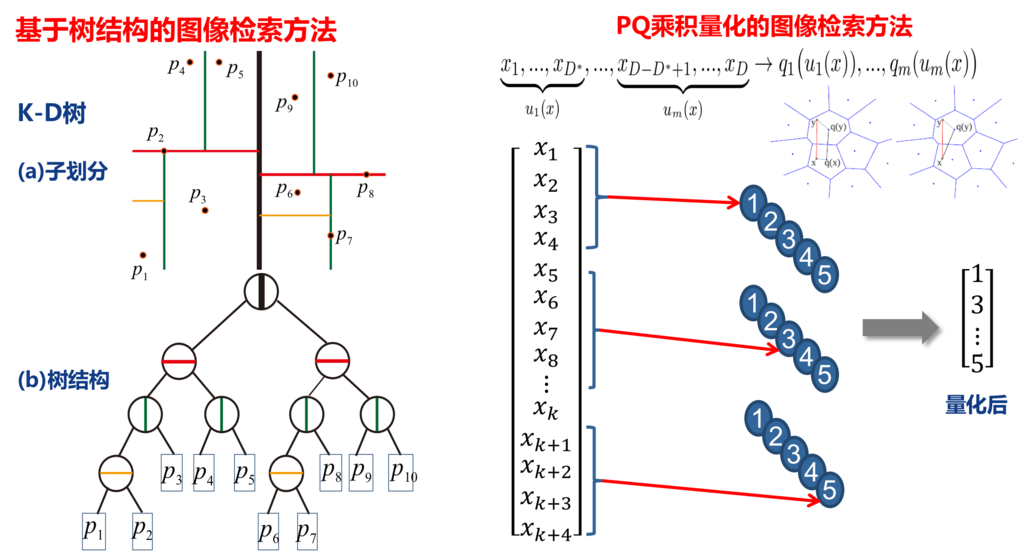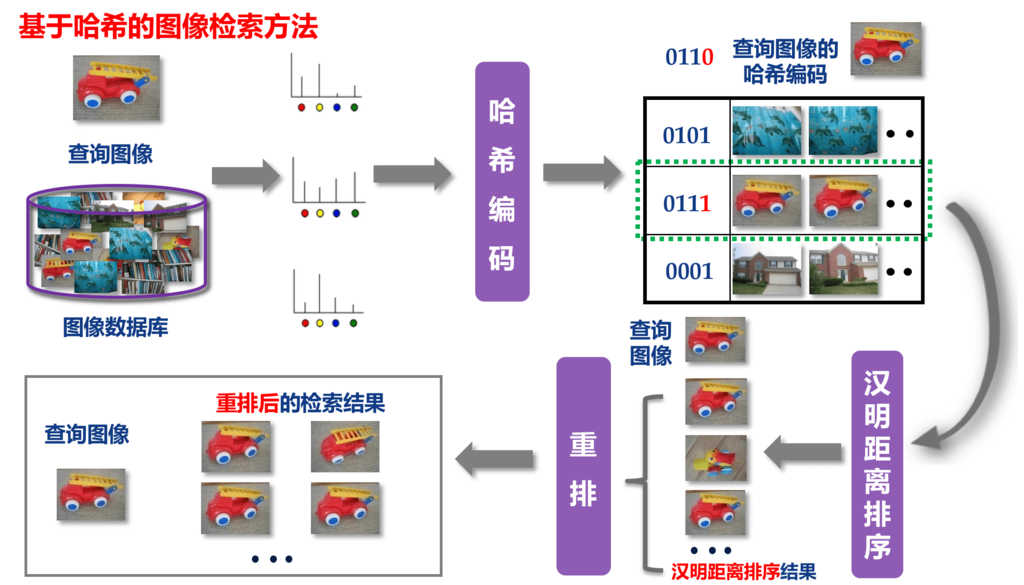• 图像数据量大
• 特征维度高
• 要求响应速度快

// Calculate cosine distance between query and data base faces
std::vector<std::pair<float, size_t> > dists_idxs;
int i = 0;
for(auto featItem: namesFeats.second){
// http://stackoverflow.com/questions/2923272/how-to-convert-vector-to-array-c
float tmp_cosine_dist = face_recognizer->CalcSimilarity(query_feat, &featItem);
dists_idxs.push_back(std::make_pair(tmp_cosine_dist, i++));
}


std::sort(dists_idxs.begin(), dists_idxs.end());
std::reverse(dists_idxs.begin(), dists_idxs.end());
for (size_t i = 0 ; i != dists_idxs.size() ; i++) {
//qDebug()<<dists_idxs[i].first<<namesFeats.first.at(dists_idxs[i].second).c_str();
QString tmpImgName = dir + '/' + namesFeats.first.at(dists_idxs[i].second).c_str();
}


• PCA降维。对于通过深度学习方式得到的特征，降维不会对信息造成大的损失，事实上在Neural codes for image retrieval这篇文章中已经指出了PCA对于深度描述子几乎不会造成损失，小白菜之前也对这样一个结论做过验证，见下图：• 使用多线程技术。在计算余弦距离的时候，使用OpenMP多线程技术，这种方式能够较大幅度的降低搜索时间，比如CPU是8线程的，则可以将原来的时间降低为原来的8分之一。

• 树结构索引方式。最经典的代表就是KD树了，小白菜用过的KD树的场合有：SIFT匹配、BoW模型。
• PQ量化以及以PQ量化进行演变的改进方法。对向量进行切分，单独做聚类进行编码。

KD树和PQ量化方式用图示意表述如下：对于KD树和PQ量化方法的优缺点，可以参阅小白菜的博文图像检索：基于内容的图像检索技术

• 基于哈希编码编码的方式。这类方法在小白菜读书的时候，为其主要研究方向。采用哈希进行图像检索的主要思想可以用下面这种图进行示意if(namesFeats.first.empty()){

// LSH搜索方案
int numFeats = (int)namesFeats.first.size();
int dim = (int)namesFeats.second.size();

// Data set parameters
uint64_t seed = 119417657;

// Common LSH parameters
int num_tables = 8;
StorageHashTable storage_hash_table = StorageHashTable::FlatHashTable;
DistanceFunction distance_function = DistanceFunction::NegativeInnerProduct;

// 转换数据类型
for (int ii = 0; ii < numFeats; ++ii) {
falconn::DenseVector<float> v = Eigen::VectorXf::Map(&namesFeats.second[ii], dim);
v.normalize(); // L2归一化
data.push_back(v);
}

// Cross polytope hashing
params_cp.dimension = dim;
params_cp.lsh_family = LSHFamily::CrossPolytope;
params_cp.distance_function = distance_function;
params_cp.storage_hash_table = storage_hash_table;
params_cp.k = 2; // 每个哈希表的哈希函数数目
params_cp.l = num_tables; // 哈希表数目
params_cp.last_cp_dimension = 2;
params_cp.num_rotations = 2;
params_cp.seed = seed ^ 833840234;

}
cptable = unique_ptr<falconn::LSHNearestNeighborTable<falconn::DenseVector<float>>>(std::move(construct_table<falconn::DenseVector<float>>(data, params_cp)));
cptable->set_num_probes(896);
qDebug() << "index build finished ...";
cptable->find_k_nearest_neighbors(q, 20, &idxCandidate);